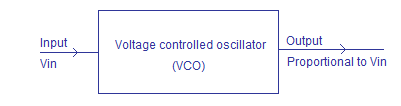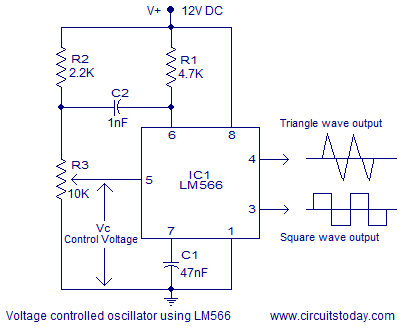## Audio

[Audio][slideshow]

### VCO (Voltage controlled oscillator).

Voltage controlled oscillator is a type of oscillator where the frequency of the output oscillations can be varied by varying the amplitude of an input voltage signal.Voltage controlled oscillators are commonly used in frequency (FM), pulse (PM) modulators and phase locked loops (PLL). Another application of the voltage controlled oscillator is the variable frequency signal generator itself.Block diagram of a typical voltage controlled oscillator is shown below.Voltage controlled oscillators can be broadly classified into linear voltage controlled oscillators and relaxation type voltage controlled oscillators. Linear voltage controlled oscillators are generally used to produce a sine wave.In such oscillators an LC tank circuit is used for producing oscillations. An active element like transistor is used for amplifying the output of the LC tank circuit, compensating the energy lost in the tank circuit and for establishing the necessary feedback conditions.Here a varactor (varicap) diode is used in place of the capacitor in the tank circuit. Varactor diode is type of semiconductor diode whose capacitance across the junction can be varied by varying the voltage across the junction.Thus by varying the voltage across the varicap diode in the tank circuit, the output frequency of the VCO can be varied.

Relaxation type voltage controlled oscillators are used to produce a sawtooth or triangular waveform. This is achieved by the gradual charging and sudden discharge of a capacitor connected appropriately to an active element (UJT, PUT etc) or a monolitic IC (LM566 etc).Now a days relaxation type VCOs are generally realized using monolithic ICs.

#### Voltage controlled oscillator using LM566 IC.

LM566 is a monolithic voltage controlled oscillator from National semiconductors. It can be used to generates square and triangle waveforms simultaneously. The frequency of the output waveform can be adjusted using an external control voltage. The output frequency can be also programmed using a set of external resistor and capacitor. Typical applications of LM566 IC are signal generators, FM modulators, FSK modulators, tone generators etc. The LM566 IC can be operated from a single supply or dual supply. While using single supply, the supply voltage range is from 10V to 24V. The IC has a very linear modulation characteristics and has excellent thermal stability. The circuit diagram of a voltage controlled oscillator using LM566 is shown in the figure below.Resistor R1 and capacitor C1 forms the timing components. Capacitor C2 is used to prevent the parasitic oscillations during VCO switching. Resistor R3 is used to provide the control voltage Vc. Triangle and square wave outputs are obtained from pins 4 and 3 respectively. Output frequency of the VCO can be obtained using the following equation:

Fout = 2.4(V+-V5) /(R1C1V+) . Where Fout is the output frequency, R1 and C1 are the timing components and V+ is the supply voltage.

[Lights][grids]